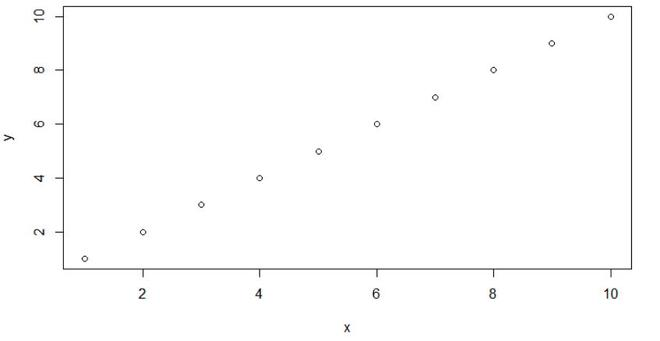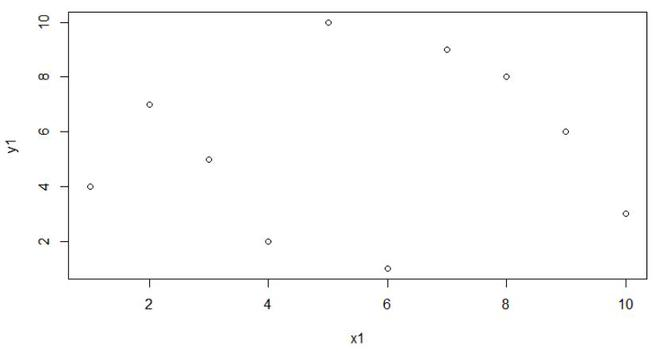# Save Plot in Data Object in Base R

• Last Updated : 31 Aug, 2021

In this article, we will be looking at the approach to save plots in data objects in R programming language.

## Using recordPlot() function

This approach is the easiest way to save any type of plot given in the data object form using the recordPlot() function. In this approach, to save the plot in the data object form user needs to all the recordPlot() functions with an object where the user needs to save the given plot, and this will automatically map the plot with the object and the given plot will be saved into the object and further when the user needs to open the plot, the user just have to call the name of the object mapped to the particular object and the plot will be displayed on the screen.

Parameters:

• load: If not NULL, a character vector of package names, which are saved as part of the recorded plot.
• attach: If not NULL, a character vector of package names, which are saved as part of the recorded plot.

Example 1: Save Plot in Data Object in R

In this example, we will be simply creating a line plot and further will be saving this plot to the data object name GFG, and then clearing the plot window and then check the saved plot in the object using the recordPlot() functions functions, we will be calling this object in the R programming language.

Input:

## R

 `x = ``c``(1, 2, 3, 4, 5,``      ``6, 7, 8, 9, 10)``y = ``c``(1, 2, 3, 4, 5,``      ``6, 7, 8, 9, 10)` `# For line Plot``plot``(x, y)` `# Saved in the object``res = ``recordPlot``() ` `# Clear the Plot Window``plot.new``()  ` `# Saved Plot``res`

Output:Example 2: Save Plot in Data Object in R

In this example, we will be creating two different line plots with different data and further will be saving this plot to the data object name GFG, GFG1 and then clearing the plot window and then check the saved plot in the object using the recordPlot() functions, we will be calling this object in the R programming language.

## R

 `x = ``c``(1, 2, 3, 4, 5,``      ``6, 7, 8, 9, 10)``y = ``c``(1, 2, 3, 4, 5,``      ``6, 7, 8, 9, 10)` `plot``(x, y)``res = ``recordPlot``()` `x1 = ``c``(5, 7, 8, 2, 9,``       ``3, 1, 10, 4, 6)``y1 = ``c``(10, 9, 8, 7, 6,``       ``5, 4, 3, 2, 1)` `plot``(x1, y1)``res2 = res = ``recordPlot``()` `plot.new``()` `res2`

Output:My Personal Notes arrow_drop_up